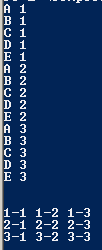# Programming Assignment #111

## Code

```//The numbers because they are in the inner loop
//Instead the letters cahange faster because they are on the inside.
//The next output is printed on the next line
//It puts them in rows of 3

public class NestingLoops
{
public static void main( String[] args )
{
// this is #1 - I'll call it "CN"
for ( int n=1; n <= 3; n++ )
{
for ( char c='A'; c <= 'E'; c++ )
{
System.out.println( c + " " + n );
}
}

System.out.println("\n");

// this is #2 - I'll call it "AB"
for ( int a=1; a <= 3; a++ )
{
for ( int b=1; b <= 3; b++ )
{
System.out.print( a + "-" + b + " " );
}
System.out.println();
// * You will add a line of code here.
}

System.out.println("\n");

}
}

```

## Outputs